Algebra 2 3-6 Guided Practice: Solving Systems Using Matrices
starstarstarstarstarstarstarstarstarstar
by Matthew Richardson
| 14 Questions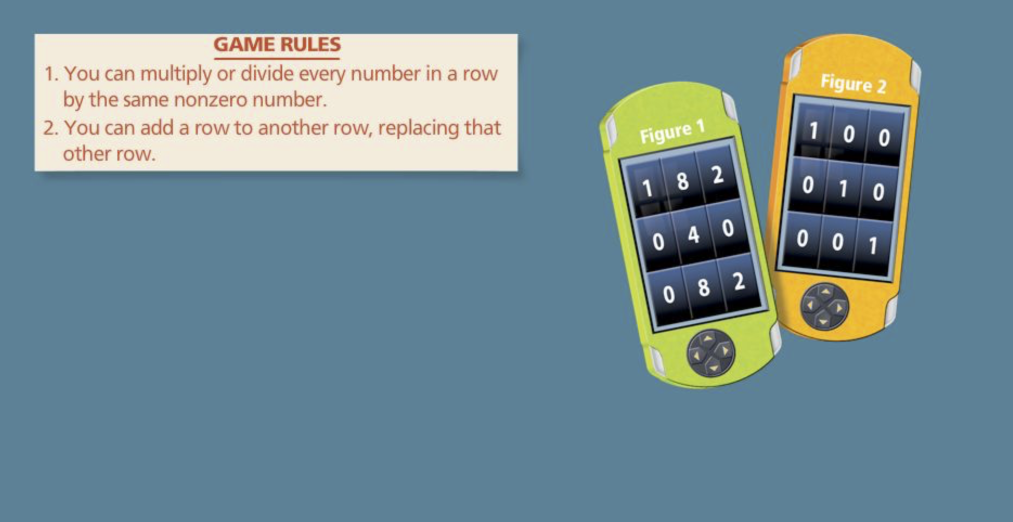1
2
1
5
Solve It! Is it possible to use the rules shown to change Figure 1 into Figure 2?
No
Yes
2
10
Solve It! Resequence the following steps to change Figure 1 into Figure 2.
1. Divide row 2 by 8 and row 3 by -2
2. Multiply row 3 by -1 and add it to row 1
3. Multiply row 2 by 2 and add it to row 3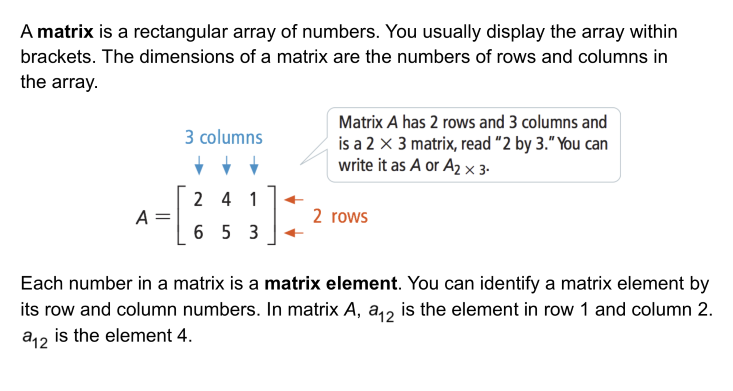3
3
10
Take Note: Define matrix.

Also, create an example 3 × 2 matrix with 6 elements on the canvas.4
4
10
Problem 1 Got It?
A
B
C
D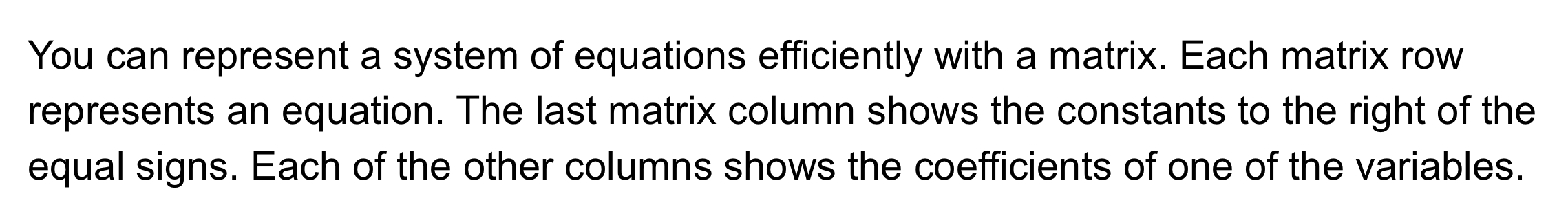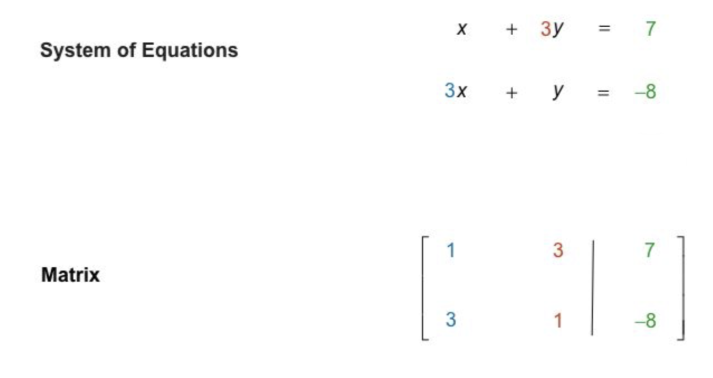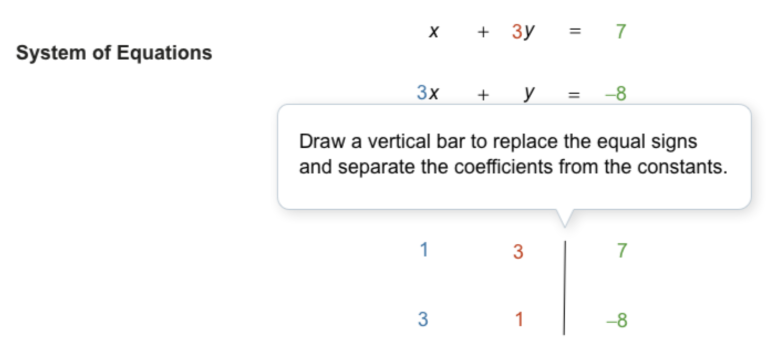5
5
10
Take Note: Summarize the process of representing systems of equations using with matrices.6
6
10
Problem 2 Got It?
A
B
C
D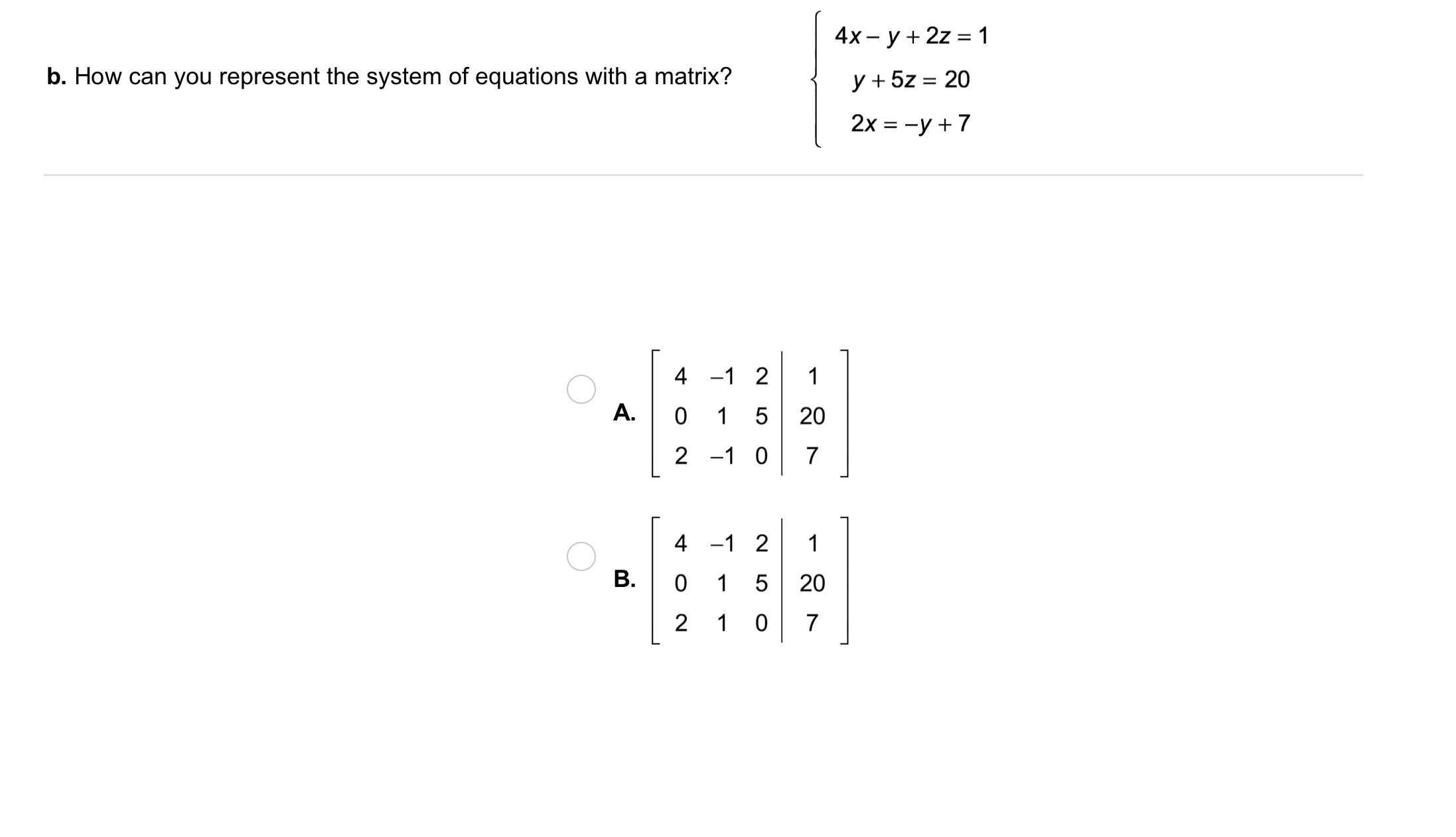7
7
10
Problem 2 Got It?
A
B
C
D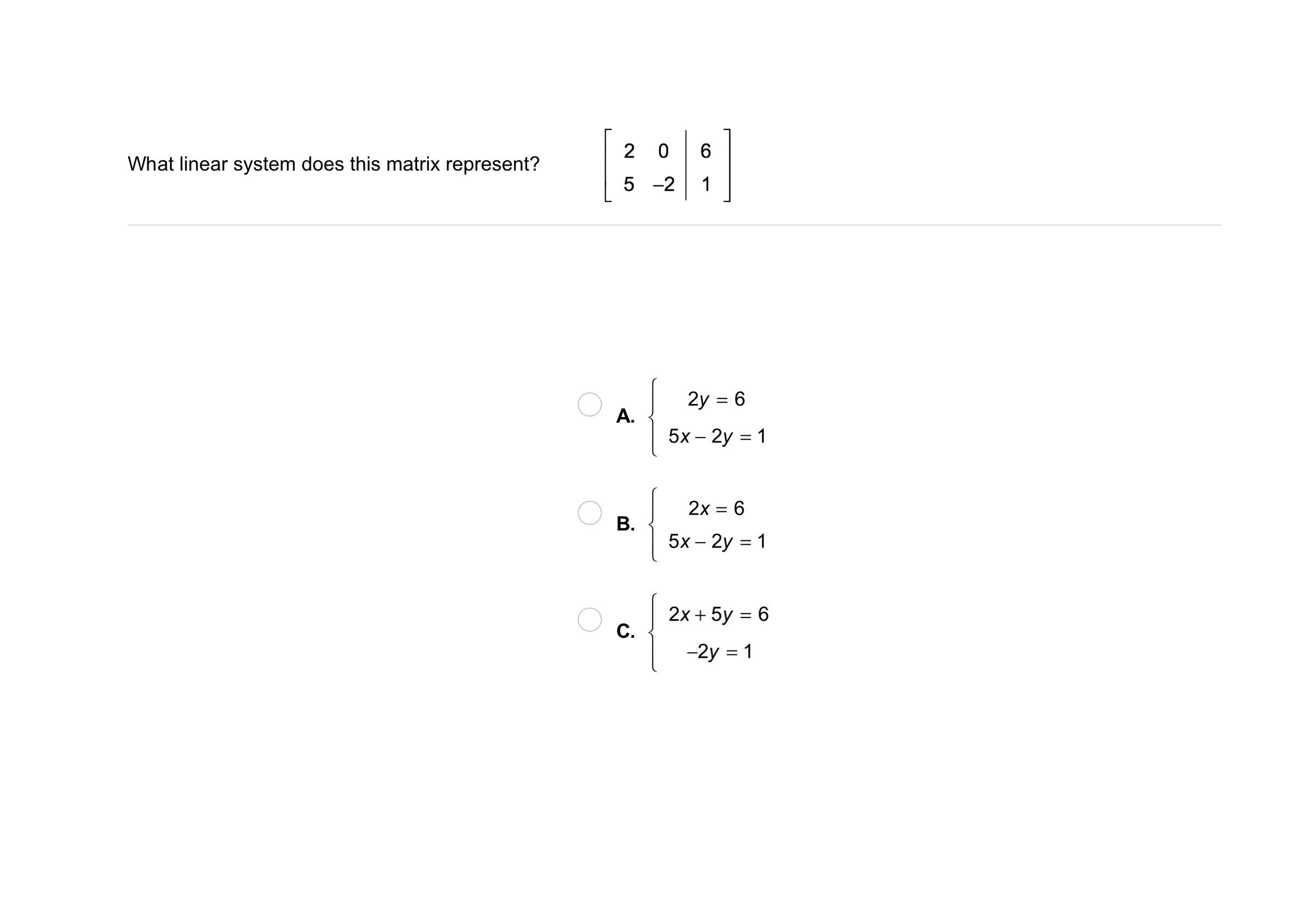8
8
10
Problem 3 Got It?
A
B
C
D9
10

Take Note: Which of the following is not considered a matrix row operation?
Multiply a column by a constant
Switch any two rows
Multiply a row by a constant
10
30
Problem 4 Got It? What is the solution of the system? Use an augmented matrix to solve and show your work on the canvas using colors that stand out. For efficiency, you may use the rectangle tool to represent matrix frames. The first frame for your augmented matrix is constructed for you as an example.
11
10
Problem 4 Got It? Resoning: Which method is more similar to solving a system using row operations: elimination or substitution? Justify your reasoning.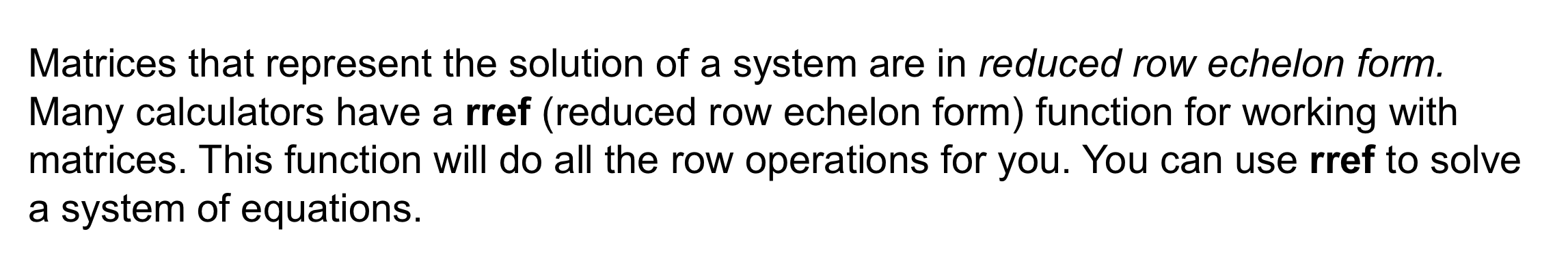A good, simple matrix rref calculator from the Linear Algebra Toolkit can be found here. Symbolab, WolframAlpha, and other utilities will also convert matrices into reduced row echelon form but may require premium access to show all row operations. Desmos is also developing a matrix calculator!12
12
10
Take Note: Summarize the process of solving a system of equations using augmented matrices and a utility with a "rref" function.13
13
10
Problem 5 Got It?
A
B
C
D14
14
10
Take Note: Summarize the mathematical content of this lesson. What topics, ideas, and vocabulary were introduced?